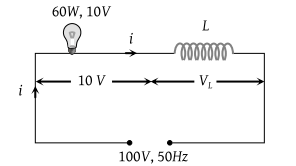NEET Questions Solved

One 10 V, 60 W bulb is to be connected to 100 V line. The required induction coil has self inductance of value (f = 50 Hz)

(1) 0.052 H

(2) 2.42 H

(3) 16.2 mH

(4) 1.62 mH

(1) Current through the bulb $i=\frac{P}{V}=\frac{60}{10}=6A$$V=\sqrt{{V}_{R}^{2}+{V}_{L}^{2}}$

${\left(100\right)}^{2}={\left(10\right)}^{2}+{V}_{L}^{2}⇒\text{\hspace{0.17em}\hspace{0.17em}}{V}_{L}=99.5\text{\hspace{0.17em}\hspace{0.17em}}Volt$

Also ${V}_{L}=i{X}_{L}=i×\left(2\pi \nu L\right)$

$⇒\text{\hspace{0.17em}}99.5=6×2×3.14×50×L$

$⇒\text{\hspace{0.17em}\hspace{0.17em}}L=0.052\text{\hspace{0.17em}\hspace{0.17em}}H$

Difficulty Level:

• 33%
• 23%
• 33%
• 12%